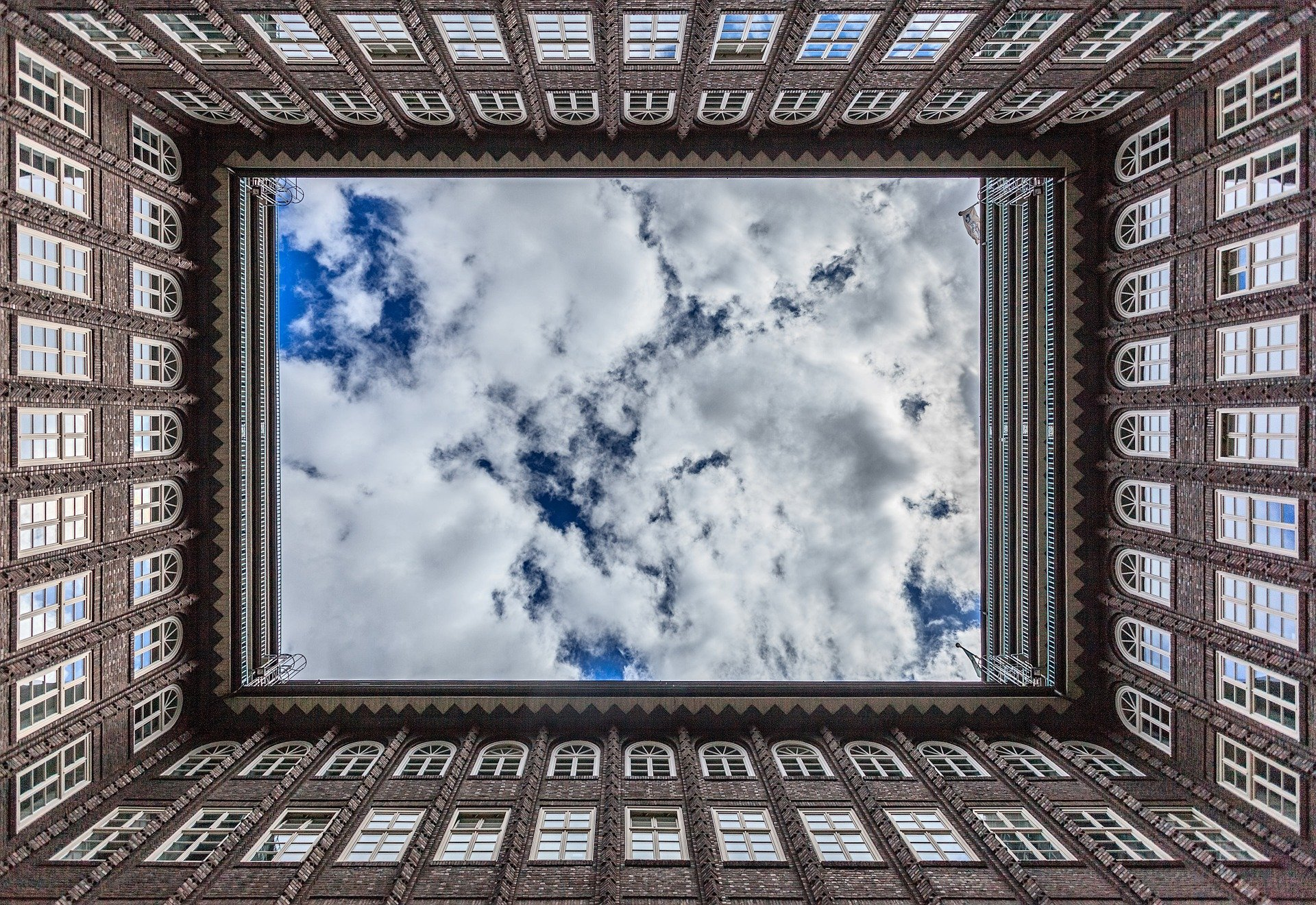What makes something symmetrical?

Symmetrical shapes or forms are the same on both sides of an imaginary line through the middle.

A square is symmetrical.

It is symmetrical because you can fold it in half, and both sides will be identical.

You can fold a rectangular piece of colored construction paper in half, horizontally or vertically. If you fold it exactly right, you’ve discovered the paper is symmetrical.

If you cut a shape from the folded construction paper, you are creating a new symmetrical form. It will have at least one line of symmetry — the fold line.

Both sides of the fold are the same. The edges line up exactly when the paper is folded in half.

Remember how that piece of paper can be folded two different ways? That means the rectangular shape of the construction paper has two lines of symmetry.

The lines of symmetry would be the fold lines.

A rectangle folded diagonally would not be symmetrical.

An oval plate is symmetrical. But it has only one line of symmetry.

A heart shape is symmetrical. It has one line of symmetry.

A paper plate is symmetrical. It has many, many lines of symmetry.

A perfect circle has an infinite (never ending) amount of lines of symmetry.

A goldfish shape is not symmetrical. It has no lines of symmetry.

Unless you are looking at it from the front.

The capital letters A, B, C, D, E, H, I, K, M, O, T, U, V, W, X, Y, are symmetrical. They each have a horizontal or a vertical line of symmetry.

The capital letters F, G, J, L, N, P, Q, R, S, Z, are not symmetrical.

The only lowercase letters that are symmetrical are c, l, o, v, w, and x.

What numbers are symmetrical? Well, the answer to that depends on what one means by a symmetrical number. But since this article is mainly talking about form or shape, then the answer is that only 0, 1, and 8 are symmetrical digits. That is to say, they are symmetrical in their form.